Maths-
General
Easy

Question

# How many different nine digit numbers can be formed from the number 223355888 by rearranging its digits so that the odd digits occupy even position ?

## 16    36    60    180Hint:

## The correct answer is: 60

### Detailed SolutionHere we need to find the total number of nine digit numbers that can be formed using the given digits i.e. 2, 2, 3, 3, 5, 5, 8, 8, 8.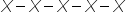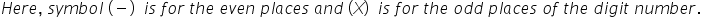The digits which are even are 2, 2, 8, 8 and 8.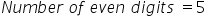The digits which are odd are 3, 3, 5 and 5.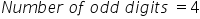We have to arrange the odd digits in even places.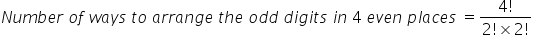On finding the value of the factorials, we get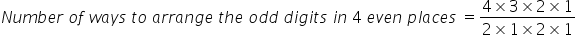On further simplification, we get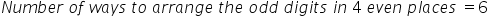Now, we have to arrange the even digits in odd places.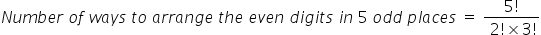On finding the value of the factorials, we get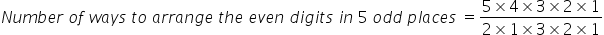On further simplification, we get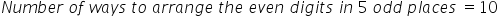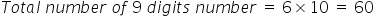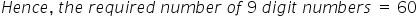Here we have obtained the total number of 9 digit numbers using the given digits. While finding the number of ways to arrange the odd digits in 5 even places, we have divided the 4! by 2! because the digit 3 were occurring two times and the digit 5 were occurring 2 times. Here we can make a mistake by conserving the number of even digits 4 and the number of odd digits 5, which will result in the wrong answer.#### With Turito Foundation.#### Get an Expert Advice From Turito.